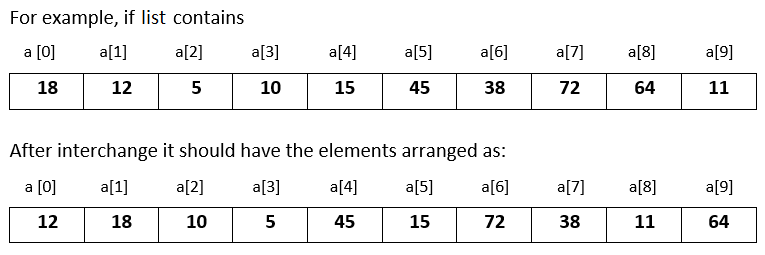# Input 10 numbers in integer list and interchange the consecutive numbers in Python

###### Lists - Question 8

In this question, we will see how to input 10 numbers in integer list and interchange the consecutive numbers in it in Python programming. To know more about lists click on the lists lesson.

Q8) Write a program in Python to input 10 numbers in integer list and interchange the consecutive numbers in it. That is, interchange a with a, a with a, a with a and so on.#### Program

``````t=0
a=list()

print('Enter 10 numbers')
for i in range(10):
a.append(int(input()))

for i in range(0,10,2):
t=a[i]
a[i]=a[i+1]
a[i+1]=t

print('\nModified list after interchanging the consecutive numbers');
for n in a:
print(n,end=' ')``````

#### Output

```Enter 10 numbers
18
12
5
10
15
45
38
72
64
11

Modified list after interchanging the consecutive numbers
12 18 10 5 45 15 72 38 11 64```